# Vue3 中的数据侦测的实现_情愿_前端开发者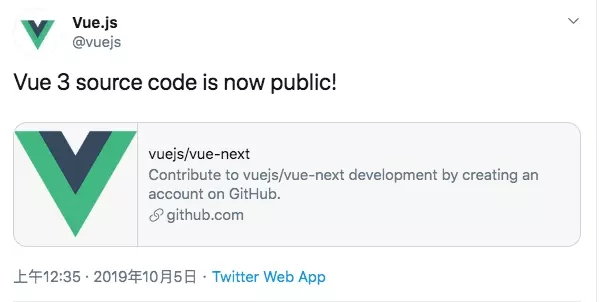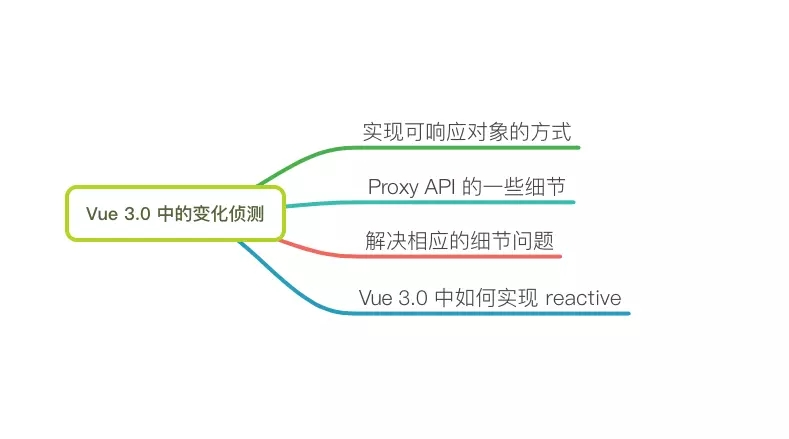vue 的核心之一就是响应式系统，通过侦测数据的变化，来驱动更新视图。

1. getter 和 setter
2. defineProperty
3. Proxy

Vue2.x 中，实现数据的可响应，需要对 Object 和 Array 两种类型采用不同的处理方式。

Object 类型通过 Object.defineProperty 将属性转换成 getter/setter ，这个过程需要递归侦测所有的对象 key，来实现深度的侦测。

Proxy API 的一些细节

Proxy API 具有更加强大的功能，相比旧的 defineProperty API ，Proxy 可以代理数组，并且 API 提供了多个 traps ，可以实现诸多功能。

```let data = { foo: 'foo' }
let p = new Proxy(data, {
return target[key]
},
console.log('set value')
target[key] = value // ?
}
})

p.foo = 123

// set value

```

```let data = [1,2,3]
let p = new Proxy(data, {
return target[key]
},
console.log('set value')
target[key] = value
}
})

p.push(4) // VM438:12 Uncaught TypeError: 'set' on proxy: trap returned falsish for property '3'

```

```let data = [1,2,3]
let p = new Proxy(data, {
return target[key]
},
console.log('set value')
target[key] = value
return true
}
})

p.push(4)

// set value // 打印2次

```

```let data = [1,2,3]
let p = new Proxy(data, {
console.log('get value:', key)
return target[key]
},
console.log('set value:', key, value)
target[key] = value
return true
}
})

p.push(1)

// get value: push
// get value: length
// set value: 3 1
// set value: length 4

```

```let data = [1,2,3]
let p = new Proxy(data, {
console.log('get value:', key)
},
console.log('set value:', key, value)
}
})

p.push(1)

// get value: push
// get value: length
// set value: 3 1
// set value: length 4

```

```let data = [1,2,3]
let p = new Proxy(data, {
console.log('get value:', key)
},
console.log('set value:', key, value)
}
})

p.unshift('a')

// get value: unshift
// get value: length
// get value: 2
// set value: 3 3
// get value: 1
// set value: 2 2
// get value: 0
// set value: 1 1
// set value: 0 a
// set value: length 4

```

```let data = { foo: 'foo', bar: { key: 1 }, ary: ['a', 'b'] }
let p = new Proxy(data, {
console.log('get value:', key)
},
console.log('set value:', key, value)
}
})

p.bar.key = 2

// get value: bar

```

```p.ary.push('c')

// get value: ary

```

```let data = { a: {b: {c: 1 } } }
let p = new Proxy(data, {
const res = Reflect.get(target, key, receiver)
return res
},
}
})

// Proxy {a: {…}}

```

```let data = { a: {b: {c: 1 } } }
let p = new Proxy(data, {
const res = Reflect.get(target, key, receiver)
console.log(res)
return res
},
}
})

// {b: {c: 1} }

```

1. 使用 Reflect 来返回 trap 默认行为
2. 对于 set 操作，可能会引发代理对象的属性更改，导致 set 执行多次
3. proxy  只能代理对象中的一层，对于对象内部的操作 set 未能感知，但是 get 会被执行

setTimeout 解决重复 trigger

```function reactive(data, cb) {
let timer = null
return new Proxy(data, {
},
clearTimeout(timer)
timer = setTimeout(() => {
cb && cb()
}, 0);
}
})
}

let ary = [1, 2]
let p = reactive(ary, () => {
console.log('trigger')
})
p.push(3)

// trigger

```

```function reactive(data, cb) {
let res = null
let timer = null

res = data instanceof Array ? []: {}

for (let key in data) {
if (typeof data[key] === 'object') {
res[key] = reactive(data[key], cb)
} else {
res[key] = data[key]
}
}

return new Proxy(res, {
get(target, key) {
return Reflect.get(target, key)
},
set(target, key, val) {
let res = Reflect.set(target, key, val)
clearTimeout(timer)
timer = setTimeout(() => {
cb && cb()
}, 0)
return res
}
})
}

let data = { foo: 'foo', bar: [1, 2] }
let p = reactive(data, () => {
console.log('trigger')
})
p.bar.push(3)

// trigger

```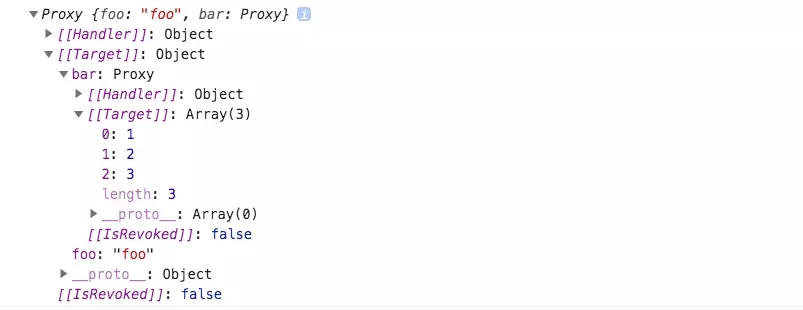Vue3 中的 reactivity

Vue3 项目结构采用了 lerna 做 monorepo 风格的代码管理，目前比较多的开源项目切换到了 monorepo 的模式，比较显著的特征是项目中会有个 packages/ 的文件夹。

Vue3 对功能做了很好的模块划分，同时使用 TS 。我们直接在 packages 中找到响应式数据的模块：```const rawToReactive = new WeakMap()
const reactiveToRaw = new WeakMap()

// utils
function isObject(val) {
return typeof val === 'object'
}

function hasOwn(val, key) {
const hasOwnProperty = Object.prototype.hasOwnProperty
return hasOwnProperty.call(val, key)
}

// traps
function createGetter() {
return function get(target, key, receiver) {
const res = Reflect.get(target, key, receiver)
return isObject(res) ? reactive(res) : res
}
}

function set(target, key, val, receiver) {
const oldValue = target[key]

val = reactiveToRaw.get(val) || val
const result = Reflect.set(target, key, val, receiver)

console.log('trigger ...')
} else if(val !== oldValue) {
console.log('trigger ...')
}

return result
}

// handler
const mutableHandlers = {
get: createGetter(),
set: set,
}

// entry
function reactive(target) {
return createReactiveObject(
target,
rawToReactive,
reactiveToRaw,
mutableHandlers,
)
}

function createReactiveObject(target, toProxy, toRaw, baseHandlers) {
let observed = toProxy.get(target)
// 原数据已经有相应的可响应数据, 返回可响应数据
if (observed !== void 0) {
return observed
}
// 原数据已经是可响应数据
if (toRaw.has(target)) {
return target
}
observed = new Proxy(target, baseHandlers)
toProxy.set(target, observed)
toRaw.set(observed, target)
return observed
}```

rawToReactive 和 reactiveToRaw 是两个弱引用的 Map 结构，这两个 Map 用来保存 原始数据 和 可响应数据 ，在函数 createReactiveObject 中，toProxy 和 toRaw 传入的便是这两个 Map 。

```let data = { foo: 'foo', ary: [1, 2] }
let r = reactive(data)
r.ary.push(3)
``````function createGetter() {
return function get(target, key, receiver) {
const res = Reflect.get(target, key, receiver)
return isObject(res) ? reactive(res) : res
}
}
```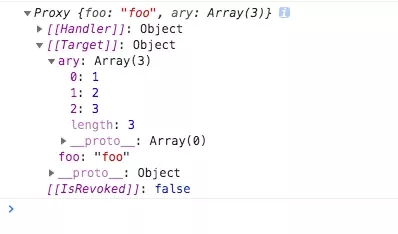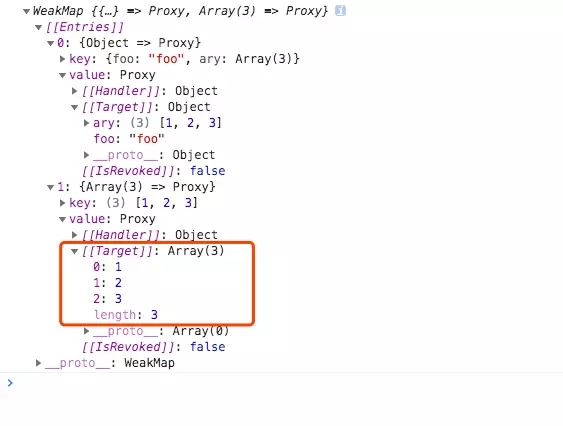```function hasOwn(val, key) {
const hasOwnProperty = Object.prototype.hasOwnProperty
return hasOwnProperty.call(val, key)
}
function set(target, key, val, receiver) {
console.log(target, key, val)
const oldValue = target[key]

val = reactiveToRaw.get(val) || val
const result = Reflect.set(target, key, val, receiver)

console.log('trigger ... is a add OperationType')
} else if(val !== oldValue) {
console.log('trigger ... is a set OperationType')
}

return result
}

```

```let data = ['a', 'b']
let r = reactive(data)
r.push('c')```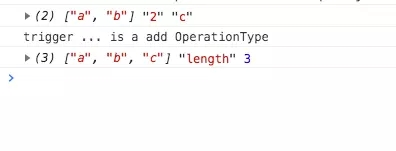r.push(‘c’) 会触发 set 执行两次，一次是值本身 ‘c’ ，一次是 length 属性设置。

» 本文来自：前端开发者 » 《Vue3 中的数据侦测的实现_情愿_前端开发者》
» 本文链接地址：https://www.rokub.com/70676.html
» 您也可以订阅本站：https://www.rokub.com
64K

• 回顶